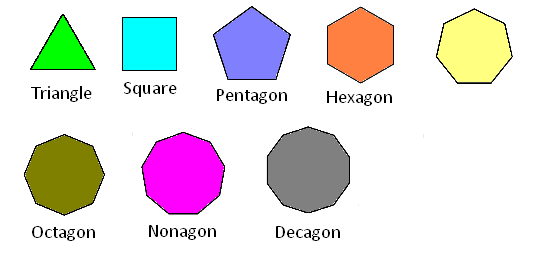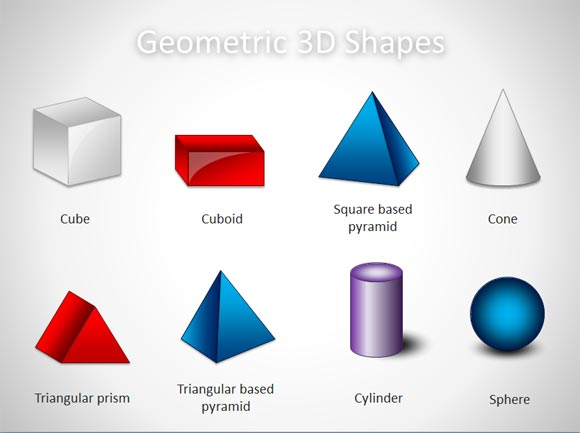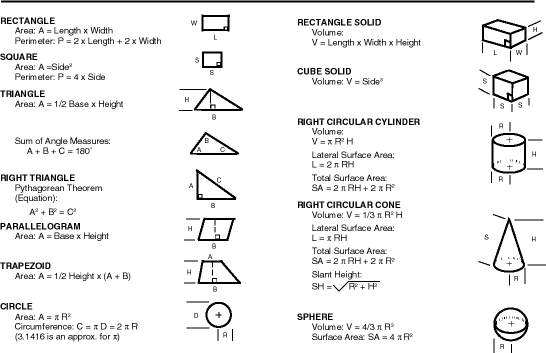Well, This topic deal's with anything classified as a shape such as: Circle,Square,Triangle,etc.. If u know the definition of Geometry that's good but for other's who don't, Geometry is the branch of mathematics concerned with the properties and relations of points, lines, surfaces, solids, and higher dimensional analogs. There a 2 way's to classified a shape.

-As a 2-D shape

• As a 3-D shapeThese images are we know as 2-D shape with only a base with length and a width. In basic math from about 3rd-7th u should learn about the basic fundamentals about these shape's and how to calculate the area or perimeter of a shape.

3-D shapes are the regular shape as a square or triangle but just 1 thing added HEIGHT!!These shape's take up more space the the formula for these shape's change's dramatically. The square u use to know has change and became a big boy.These are some formulas of 2-D and 3-D shape's. (Sorry for such small Images) Mostly for like a square or rectangle the formula hasn't really change but just added height but, once u hit a circle now u talking about my favorite subject MATH!!

• As u can see the formula for a circle or the shape we know as 3-D a sphere is 4/3pi^r^2 which this is a complex formula to learn but, is hard to work with!!!

Okay this is all for this topic I will do another Discussion but Pt.2 of Geometry which will be released on Friday, March 31 2017.

Until then, Keep solving equations and the fundamental's of Math

-P.S

Pt.2 of this topic I will discuss more of the Formula of a Circle and I will show some example's as well as discussing how to solve for a missing variable such as pi or the radiusNote by Noah Smalls
4 years, 4 months ago

This discussion board is a place to discuss our Daily Challenges and the math and science related to those challenges. Explanations are more than just a solution — they should explain the steps and thinking strategies that you used to obtain the solution. Comments should further the discussion of math and science.

When posting on Brilliant:

• Use the emojis to react to an explanation, whether you're congratulating a job well done , or just really confused .
• Ask specific questions about the challenge or the steps in somebody's explanation. Well-posed questions can add a lot to the discussion, but posting "I don't understand!" doesn't help anyone.
• Try to contribute something new to the discussion, whether it is an extension, generalization or other idea related to the challenge.

MarkdownAppears as
*italics* or _italics_ italics
**bold** or __bold__ bold
- bulleted- list
• bulleted
• list
1. numbered2. list
1. numbered
2. list
Note: you must add a full line of space before and after lists for them to show up correctly
paragraph 1paragraph 2

paragraph 1

paragraph 2

[example link](https://brilliant.org)example link
> This is a quote
This is a quote
    # I indented these lines
# 4 spaces, and now they show
# up as a code block.

print "hello world"
# I indented these lines
# 4 spaces, and now they show
# up as a code block.

print "hello world"
MathAppears as
Remember to wrap math in $$ ... $$ or $ ... $ to ensure proper formatting.
2 \times 3 $2 \times 3$
2^{34} $2^{34}$
a_{i-1} $a_{i-1}$
\frac{2}{3} $\frac{2}{3}$
\sqrt{2} $\sqrt{2}$
\sum_{i=1}^3 $\sum_{i=1}^3$
\sin \theta $\sin \theta$
\boxed{123} $\boxed{123}$

Sort by:

@Noah Smalls Do you love Geometry? (This one was b/c I love it more than anything else....) My actual question: Are you an IMO aspirant, just looking for IMO or not ?

- 4 years, 3 months ago